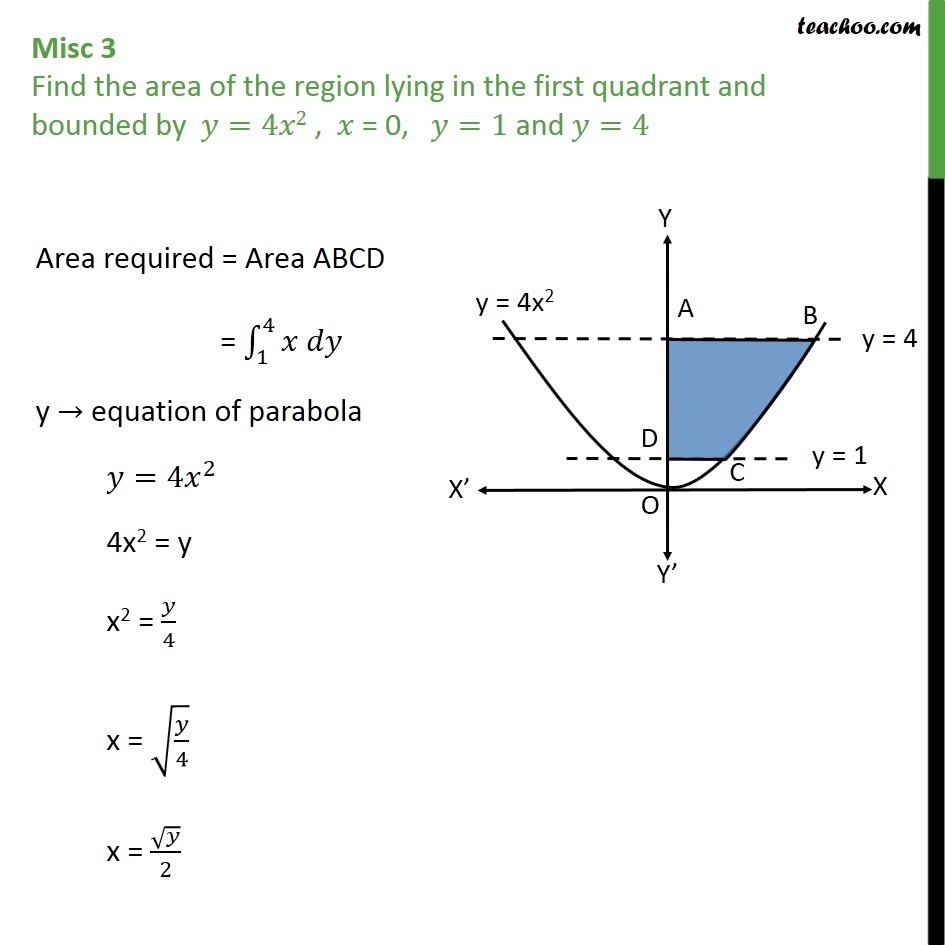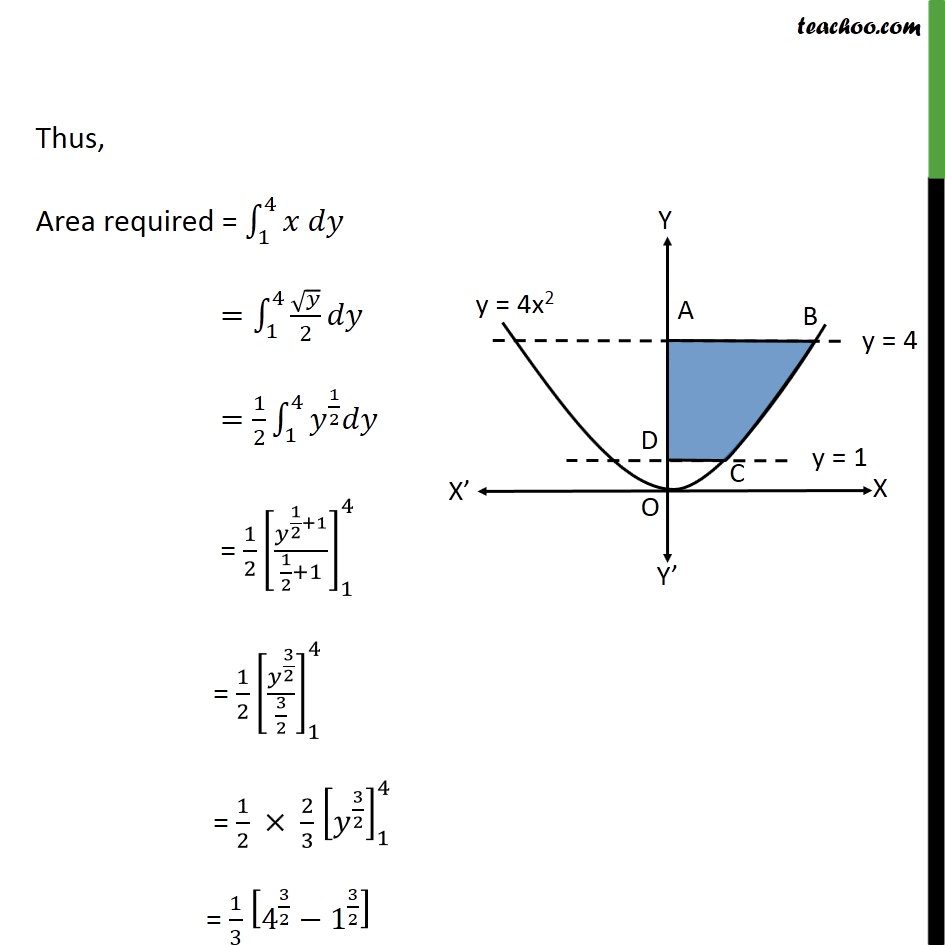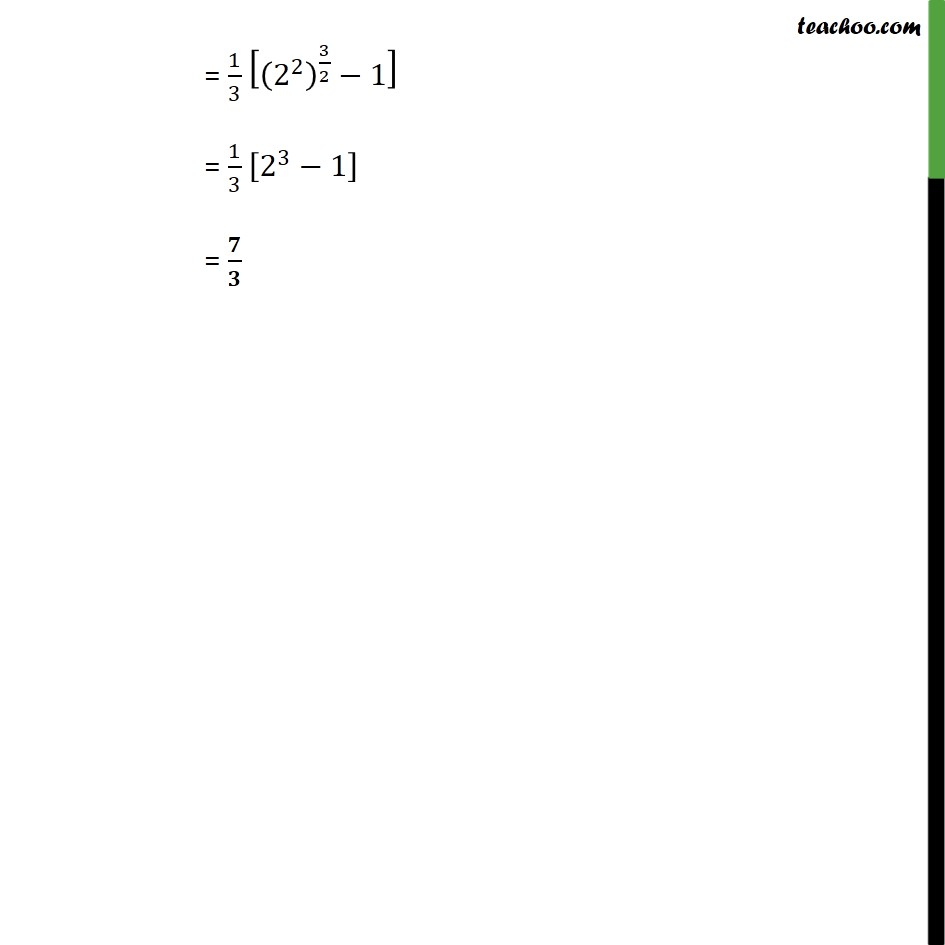Area bounded by curve and horizontal or vertical line

Chapter 8 Class 12 Application of Integrals
Concept wiseLearn in your speed, with individual attention - Teachoo Maths 1-on-1 Class

### Transcript

Question 2 Find the area of the region lying in the first quadrant and bounded by =4 2 , = 0, =1 and =4 Area required = Area ABCD = 1 4 y equation of parabola = 4 2 4x2 = y x2 = 4 x = 4 x = 2 Thus, Area required = 1 4 = 1 2 4 2 =4 3 3 1 2 = 1 3 2 3 1 3 = 1 3 8 1 = 7 3 Thus, Area required = 1 4 = 1 4 2 = 1 2 1 4 1 2 = 1 2 1 2 +1 1 2 +1 1 4 = 1 2 3 2 3 2 1 4 = 1 2 2 3 3 2 1 4 = 1 3 4 3 2 1 3 2 = 2 3 = 1 3 2 2 3 2 1 = 1 3 2 3 1 =